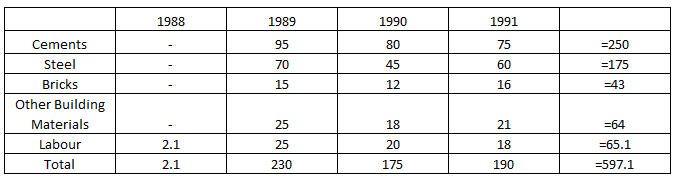### CAT 1990 Question Paper Question 63

Instructions

The table below shows the estimated cost (in Rs. Lakh) of a project of laying a railway line between two places.

Question 63

# What is the approximate ratio of the total cost of materials for all the years bear to the total labour cost?

SolutionTotal material for all the years will be = 250+175+43+64 = 532
Total labour cost for all the years = 65.1
Hence ratio will be $$\frac{65.1}{532}$$ nearly equal to 8:1.

• All Quant CAT Formulas and shortcuts PDF
• 30+ CAT previous papers with solutions PDF